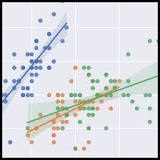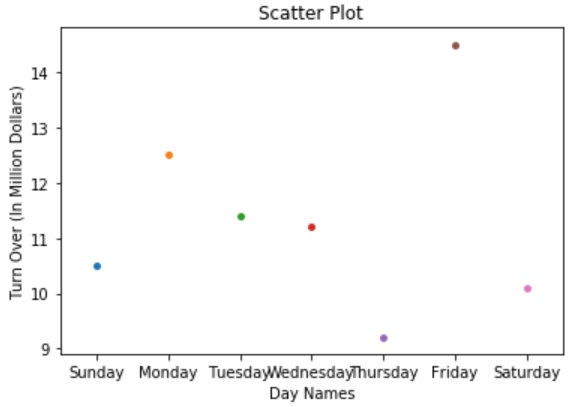# Plotting graph using seaborn in python.

Plotly's Python graphing library makes interactive, publication-quality graphs online. this graph is mainly used when we want to make line plots, scatter plots, area charts, bar charts, error bars, box plots, histograms, heatmaps, subplots, multiple-axes, polar charts, and bubble charts.

Seaborn is a library for making statistical graphics in Python. It is built on top of matplotlib and it is integrated with pandas data structures.1. We import seaborn, which is the only library necessary for this simple example.

import seaborn as sns


2. We apply the default default seaborn theme, scaling, and color palette.

sns.set()


3. We load one of the example datasets.

tips = sns.load_dataset("tips")


4. We draw a faceted scatter plot with multiple semantic variables.

## Example Code

# This Python program will illustrate scatter plot with Seaborn
# importing modules
import matplotlib.pyplot as plt
import seaborn as sns
# values for x-axis
x=['Sunday', 'Monday', 'Tuesday', 'Wednesday', 'Thursday', 'Friday', 'Saturday']
# valueds for y-axis
y=[10.5, 12.5, 11.4, 11.2, 9.2, 14.5, 10.1]
# plotting with seaborn
my_plot = sns.stripplot(x, y);
# assigning x-axis and y-axis labels
my_plot.set(xlabel ='Day Names', ylabel ='Turn Over (In Million Dollars)')
# assigning plot title
plt.title('Scatter Plot');
# function to show plot
plt.show()


## Output